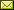## Learning Markov Decision Processes for Model Checking

 Hua Mao (AAU) Yingke Chen (AAU) Manfred Jaeger (AAU) Thomas D. Nielsen (AAU) Kim G. Larsen (AAU) Brian Nielsen (AAU)

 Constructing an accurate system model for formal model verification can be both resource demanding and time-consuming. To alleviate this shortcoming, algorithms have been proposed for automatically learning system models based on observed system behaviors. In this paper we extend the algorithm on learning probabilistic automata to reactive systems, where the observed system behavior is in the form of alternating sequences of inputs and outputs. We propose an algorithm for automatically learning a deterministic labeled Markov decision process model from the observed behavior of a reactive system. The proposed learning algorithm is adapted from algorithms for learning deterministic probabilistic finite automata, and extended to include both probabilistic and nondeterministic transitions. The algorithm is empirically analyzed and evaluated by learning system models of slot machines. The evaluation is performed by analyzing the probabilistic linear temporal logic properties of the system as well as by analyzing the schedulers, in particular the optimal schedulers, induced by the learned models.

In Uli Fahrenberg, Axel Legay and Claus Thrane: Proceedings Quantities in Formal Methods (QFM 2012), Paris, France, 28 August 2012, Electronic Proceedings in Theoretical Computer Science 103, pp. 49–63.
Published: 14th December 2012.

 ArXived at: https://dx.doi.org/10.4204/EPTCS.103.6 bibtex PDF
References in reconstructed bibtex, XML and HTML format (approximated).
 Comments and questions to:eptcs@eptcs.org For website issues:webmaster@eptcs.org